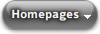Parc SystemsHistoryLatest News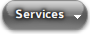TestingTrainingResaleOther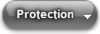GeneratorTransformerBus BarMotorAuxiliary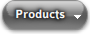RelaysTest equipmentIDMT CurvesThermal curves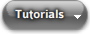Relay TestingTest Equipment UseAutomatic Test Equipment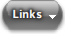CEE Relays LtdABBSiemensGeneral ElectricSchniederArevaR & B SwitchgearP & BISAOmicronAVO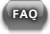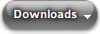BrochuresDrawingsTest Instructions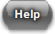MembersSign Up
Html Popup Menu by Vista-Buttons.com v5.7

# The thermal trip time calculatorThe calculated thermal trip times on this page are based on the theory that most motor trip times follow the exponential law 1 - 1/ex.
That being the case the trip time can be calculated from any prior thermal state using the following formula.
T = Tau x loge((M² - P²)/(M² - O²))
Where
• Tau = The time for the motor to reach 63.21 percent hot under normal FLC conditions
• M = The multiple of FLC applied
• P = The prior thermal state of the motor before the fault current was applied
• O = The max overload setting of the relay before a trip will occur i.e. The permanent overload withstand
For example, for a 60A motor if a 120A fault current was simulated then M would equal 2.
If needing to calculate the trip time from cold then P would equal zero.
If needing to calculate the trip time from 100% hot then P would equal 1
If the motor has a permanent overload setting of 7 percent (i.e. you can run the motor up to 107 percent FLC without causing a trip) then O would equal 1.07
Remember that all values entered into the calculator should be per unit values and NOT percentages. (i.e. 80 percent = 0.8 as a per unit value)

M =  Tau =  P =  O =

## New website feature! Calculate your relay trip times

Why not use our new calculator feature to calculate the trip times of your overcurrent or thermal relays. Tripping curves based on the IEC formulas for all types of inverse curves.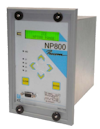Check out the latest NP800 range from CEECheck out the latest Micom range from Schneider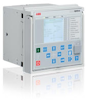Check out the latest REF range from ABBCheck other switchgear services here